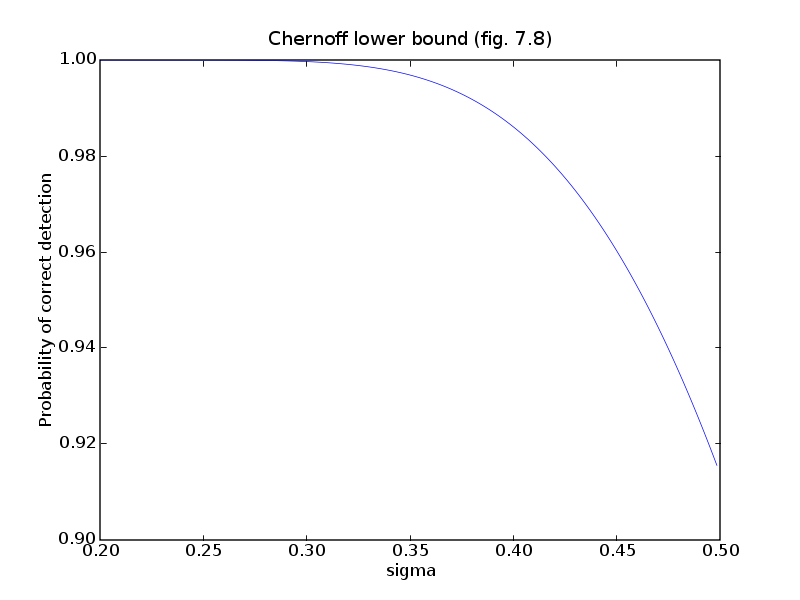# Chernoff lower bound (fig. 7.8)¶`source code`

```# Figure 7.8, page 384.
# Chernoff lower bound.

from cvxopt import matrix, mul, exp, normal, solvers, blas
solvers.options['show_progress'] = False

# Extreme points and inequality description of Voronoi region around
# first symbol (at the origin).
m = 6
V = matrix([ 1.0,  1.0,
-1.0,  2.0,
-2.0,  1.0,
-2.0, -1.0,
0.0, -2.0,
1.5, -1.0,
1.0,  1.0 ], (2,m+1))

# A and b are lists with the inequality descriptions of the regions.
A = [ matrix( [-(V[1,:m] - V[1,1:]), V[0,:m] - V[0,1:]] ).T ]
b = [ mul(A, V[:,:m].T) * matrix(1.0, (2,1)) ]

# List of symbols.
C = [ matrix(0.0, (2,1)) ] + \
[ 2.0 * b[k] / blas.nrm2(A[k,:])**2 * A[k,:].T for k in
range(m) ]

# Voronoi set around C
A += [ matrix(0.0, (3,2)) ]
b += [ matrix(0.0, (3,1)) ]
A[0,:] = -A[0,:]
b = -b
A[1,:] = (C[m] - C).T
b = 0.5 * A[1,:] * ( C[m] + C )
A[2,:] = (C - C).T
b = 0.5 * A[2,:] * ( C + C )

# Voronoi set around C, ..., C
for k in range(2, 6):
A += [ matrix(0.0, (3,2)) ]
b += [ matrix(0.0, (3,1)) ]
A[k][0,:] = -A[k-1,:]
b[k] = -b[k-1]
A[k][1,:] = (C[k-1] - C[k]).T
b[k] = 0.5 * A[k][1,:] * ( C[k-1] + C[k] )
A[k][2,:] = (C[k+1] - C[k]).T
b[k] = 0.5 * A[k][2,:] * ( C[k+1] + C[k] )

# Voronoi set around C
A += [ matrix(0.0, (3,2)) ]
b += [ matrix(0.0, (3,1)) ]
A[0,:] = -A[5,:]
b = -b
A[1,:] = (C - C).T
b = 0.5 * A[1,:] * ( C + C )
A[2,:] = (C - C).T
b = 0.5 * A[2,:] * ( C + C )

# For regions k=1, ..., 6, let pk be the optimal value of
#
#     minimize    x'*x
#     subject to  A*x <= b.
#
# The Chernoff upper bound is  1.0 - sum exp( - pk / (2 sigma^2)).

P = matrix([1.0, 0.0, 0.0, 1.0], (2,2))
q = matrix(0.0, (2,1))
optvals = matrix([ blas.nrm2( solvers.qp(P, q, A[k], b[k] )['x'] )**2
for k in range(1,7) ])
nopts = 200
sigmas = 0.2 + (0.5 - 0.2)/nopts * matrix(list(range(nopts)), tc='d')
bnds = [ 1.0 - sum( exp( - optvals / (2*sigma**2) )) for sigma
in sigmas]

try: import pylab
except ImportError: pass
else:
pylab.figure(facecolor='w')
pylab.plot(sigmas, bnds, '-')
pylab.axis([0.2, 0.5, 0.9, 1.0])
pylab.title('Chernoff lower bound (fig. 7.8)')
pylab.xlabel('sigma')
pylab.ylabel('Probability of correct detection')
pylab.show()

if 0:  # uncomment out for the Monte Carlo estimation.
N = 100000
mcest = []
ones = matrix(1.0, (1,m))
for sigma in sigmas:
X = sigma * normal(2, N)
S = b[:,N*] - A*X
S = ones * (S - abs(S))
mcest += [ N - len(filter(lambda x: x < 0.0, S)) ]

pylab.figure(facecolor='w')
pylab.plot(sigmas, bnds, '-', sigmas, (1.0/N)*matrix(mcest), '--')
pylab.plot(sigmas, bnds, '-')
pylab.axis([0.2, 0.5, 0.9, 1.0])
pylab.title('Chernoff lower bound (fig. 7.8)')
pylab.xlabel('sigma')
pylab.ylabel('Probability of correct detection')
pylab.show()
```